# 5.3 Graphs of polynomial functions  (Page 9/13)

 Page 9 / 13

$f\left(x\right)={x}^{5}-2x,\text{\hspace{0.17em}}$ between $\text{\hspace{0.17em}}x=1\text{\hspace{0.17em}}$ and $\text{\hspace{0.17em}}x=2.$

$f\left(x\right)=-{x}^{4}+4,\text{\hspace{0.17em}}$ between $\text{\hspace{0.17em}}x=1\text{\hspace{0.17em}}$ and $\text{\hspace{0.17em}}x=3$ .

$f\left(1\right)=3\text{\hspace{0.17em}}$ and $\text{\hspace{0.17em}}f\left(3\right)=–77.\text{\hspace{0.17em}}$ Sign change confirms.

$f\left(x\right)=-2{x}^{3}-x,\text{\hspace{0.17em}}$ between $\text{\hspace{0.17em}}x=–1\text{\hspace{0.17em}}$ and $\text{\hspace{0.17em}}x=1.$

$f\left(x\right)={x}^{3}-100x+2,\text{\hspace{0.17em}}$ between $\text{\hspace{0.17em}}x=0.01\text{\hspace{0.17em}}$ and $\text{\hspace{0.17em}}x=0.1$

$f\left(0.01\right)=1.000001\text{\hspace{0.17em}}$ and $\text{\hspace{0.17em}}f\left(0.1\right)=–7.999.\text{\hspace{0.17em}}$ Sign change confirms.

For the following exercises, find the zeros and give the multiplicity of each.

$f\left(x\right)={\left(x+2\right)}^{3}{\left(x-3\right)}^{2}$

$f\left(x\right)={x}^{2}{\left(2x+3\right)}^{5}{\left(x-4\right)}^{2}$

0 with multiplicity 2, $\text{\hspace{0.17em}}-\frac{3}{2}\text{\hspace{0.17em}}$ with multiplicity 5, 4 with multiplicity 2

$f\left(x\right)={x}^{3}{\left(x-1\right)}^{3}\left(x+2\right)$

$f\left(x\right)={x}^{2}\left({x}^{2}+4x+4\right)$

0 with multiplicity 2, –2 with multiplicity 2

$f\left(x\right)={\left(2x+1\right)}^{3}\left(9{x}^{2}-6x+1\right)$

$f\left(x\right)={\left(3x+2\right)}^{5}\left({x}^{2}-10x+25\right)$

$-\frac{2}{3}\text{\hspace{0.17em}}\text{with}\text{\hspace{0.17em}}\text{multiplicity}\text{\hspace{0.17em}}5\text{,}\text{\hspace{0.17em}}5\text{\hspace{0.17em}}\text{with}\text{\hspace{0.17em}}\text{multiplicity}\text{\hspace{0.17em}}\text{2}$

$f\left(x\right)=x\left(4{x}^{2}-12x+9\right)\left({x}^{2}+8x+16\right)$

$f\left(x\right)={x}^{6}-{x}^{5}-2{x}^{4}$

$\text{0}\text{\hspace{0.17em}}\text{with}\text{\hspace{0.17em}}\text{multiplicity}\text{\hspace{0.17em}}4\text{,}\text{\hspace{0.17em}}2\text{\hspace{0.17em}}\text{with}\text{\hspace{0.17em}}\text{multiplicity}\text{\hspace{0.17em}}1\text{,}\text{\hspace{0.17em}}–\text{1}\text{\hspace{0.17em}}\text{with}\text{\hspace{0.17em}}\text{multiplicity}\text{\hspace{0.17em}}1$

$f\left(x\right)=3{x}^{4}+6{x}^{3}+3{x}^{2}$

$f\left(x\right)=4{x}^{5}-12{x}^{4}+9{x}^{3}$

$\frac{3}{2}\text{\hspace{0.17em}}$ with multiplicity 2, 0 with multiplicity 3

$f\left(x\right)=2{x}^{4}\left({x}^{3}-4{x}^{2}+4x\right)$

$f\left(x\right)=4{x}^{4}\left(9{x}^{4}-12{x}^{3}+4{x}^{2}\right)$

$\text{0}\text{\hspace{0.17em}}\text{with}\text{\hspace{0.17em}}\text{multiplicity}\text{\hspace{0.17em}}6\text{,}\text{\hspace{0.17em}}\frac{2}{3}\text{\hspace{0.17em}}\text{with}\text{\hspace{0.17em}}\text{multiplicity}\text{\hspace{0.17em}}2$

## Graphical

For the following exercises, graph the polynomial functions. Note $\text{\hspace{0.17em}}x\text{-}$ and $\text{\hspace{0.17em}}y\text{-}$ intercepts, multiplicity, and end behavior.

$f\left(x\right)={\left(x+3\right)}^{2}\left(x-2\right)$

$g\left(x\right)=\left(x+4\right){\left(x-1\right)}^{2}$

x -intercepts, $\left(1, 0\right)$ with multiplicity 2, with multiplicity 1, $y\text{-}$ intercept As $\phantom{\rule{0.2em}{0ex}}x\to -\infty ,\phantom{\rule{0.2em}{0ex}}f\left(x\right)\to -\infty ,\text{\hspace{0.17em}}\text{as}\phantom{\rule{0.2em}{0ex}}x\to \infty ,\phantom{\rule{0.2em}{0ex}}f\left(x\right)\to \infty .$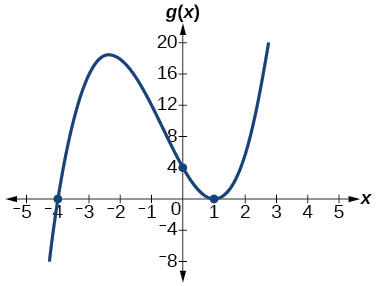$h\left(x\right)={\left(x-1\right)}^{3}{\left(x+3\right)}^{2}$

$k\left(x\right)={\left(x-3\right)}^{3}{\left(x-2\right)}^{2}$

x -intercepts $\text{\hspace{0.17em}}\left(3,0\right)\text{\hspace{0.17em}}$ with multiplicity 3, $\text{\hspace{0.17em}}\left(2,0\right)\text{\hspace{0.17em}}$ with multiplicity 2, $\text{\hspace{0.17em}}y\text{-}$ intercept $\text{\hspace{0.17em}}\left(0,–108\right).\text{\hspace{0.17em}}$ As $x\to -\infty ,\phantom{\rule{0.2em}{0ex}}f\left(x\right)\to -\infty ,\phantom{\rule{0.2em}{0ex}}\text{as}\phantom{\rule{0.2em}{0ex}}x\to \infty ,\phantom{\rule{0.2em}{0ex}}f\left(x\right)\to \infty .$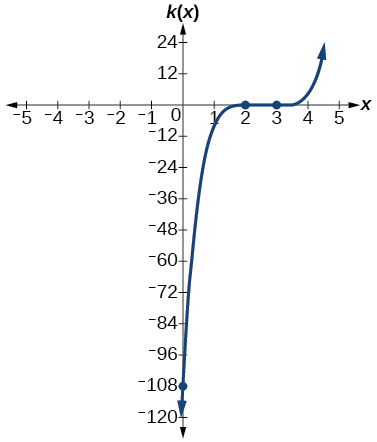$m\left(x\right)=-2x\left(x-1\right)\left(x+3\right)$

$n\left(x\right)=-3x\left(x+2\right)\left(x-4\right)$

x -intercepts with multiplicity 1, $\text{\hspace{0.17em}}y\text{-}$ intercept As $x\to -\infty ,\phantom{\rule{0.2em}{0ex}}f\left(x\right)\to \infty ,\phantom{\rule{0.2em}{0ex}}\text{as}\phantom{\rule{0.2em}{0ex}}x\to \infty ,\phantom{\rule{0.2em}{0ex}}f\left(x\right)\to -\infty .$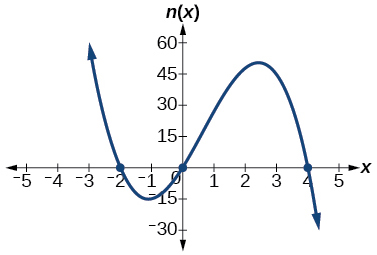For the following exercises, use the graphs to write the formula for a polynomial function of least degree.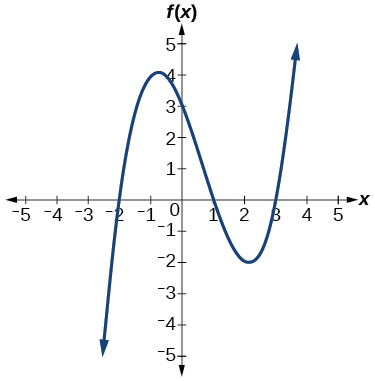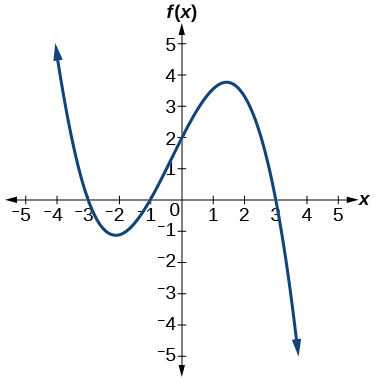$f\left(x\right)=-\frac{2}{9}\left(x-3\right)\left(x+1\right)\left(x+3\right)$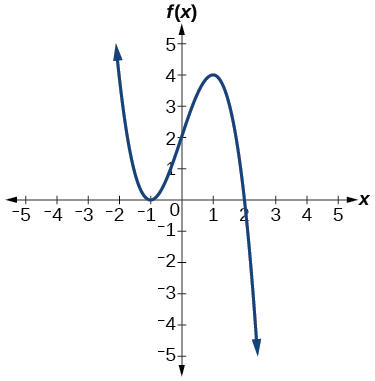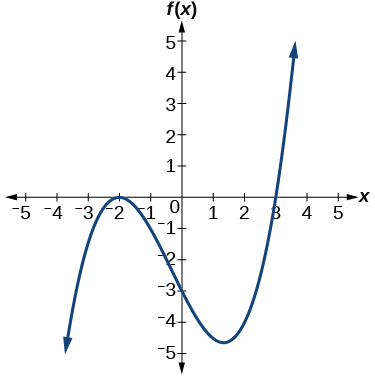$f\left(x\right)=\frac{1}{4}{\left(x+2\right)}^{2}\left(x-3\right)$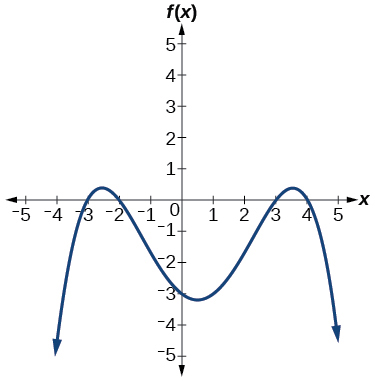For the following exercises, use the graph to identify zeros and multiplicity.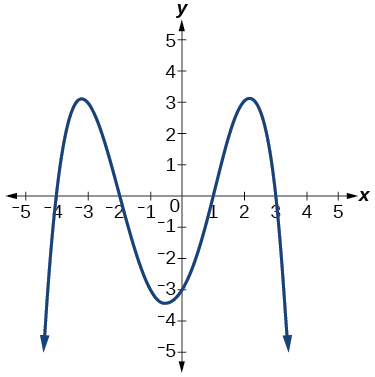–4, –2, 1, 3 with multiplicity 1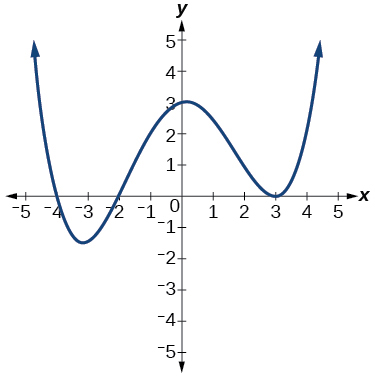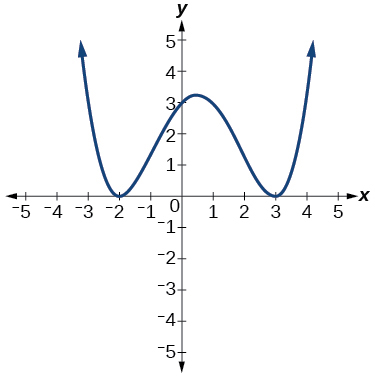–2, 3 each with multiplicity 2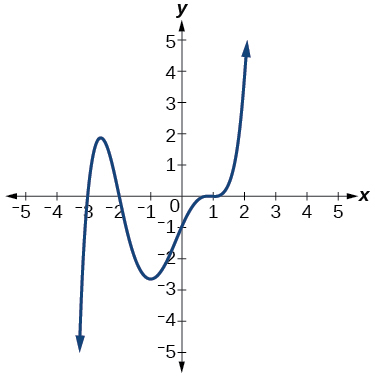For the following exercises, use the given information about the polynomial graph to write the equation.

Degree 3. Zeros at $\text{\hspace{0.17em}}x=–2,$ $\text{\hspace{0.17em}}x=1,\text{\hspace{0.17em}}$ and $\text{\hspace{0.17em}}x=3.\text{\hspace{0.17em}}$ y -intercept at $\text{\hspace{0.17em}}\left(0,–4\right).$

$f\left(x\right)=-\frac{2}{3}\left(x+2\right)\left(x-1\right)\left(x-3\right)$

Degree 3. Zeros at $\text{\hspace{0.17em}}x=\text{–5,}$ $\text{\hspace{0.17em}}x=–2,$ and $\text{\hspace{0.17em}}x=1.\text{\hspace{0.17em}}$ y -intercept at $\text{\hspace{0.17em}}\left(0,6\right)$

Degree 5. Roots of multiplicity 2 at $\text{\hspace{0.17em}}x=3\text{\hspace{0.17em}}$ and $\text{\hspace{0.17em}}x=1\text{\hspace{0.17em}}$ , and a root of multiplicity 1 at $\text{\hspace{0.17em}}x=–3.\text{\hspace{0.17em}}$ y -intercept at $\text{\hspace{0.17em}}\left(0,9\right)$

$f\left(x\right)=\frac{1}{3}{\left(x-3\right)}^{2}{\left(x-1\right)}^{2}\left(x+3\right)$

Degree 4. Root of multiplicity 2 at $\text{\hspace{0.17em}}x=4,\text{\hspace{0.17em}}$ and a roots of multiplicity 1 at $\text{\hspace{0.17em}}x=1\text{\hspace{0.17em}}$ and $\text{\hspace{0.17em}}x=–2.\text{\hspace{0.17em}}$ y -intercept at $\text{\hspace{0.17em}}\left(0,\text{–}3\right).$

Degree 5. Double zero at $\text{\hspace{0.17em}}x=1,\text{\hspace{0.17em}}$ and triple zero at $\text{\hspace{0.17em}}x=3.\text{\hspace{0.17em}}$ Passes through the point $\text{\hspace{0.17em}}\left(2,15\right).$

$f\left(x\right)=-15{\left(x-1\right)}^{2}{\left(x-3\right)}^{3}$

Degree 3. Zeros at $\text{\hspace{0.17em}}x=4,$ $\text{\hspace{0.17em}}x=3,$ and $\text{\hspace{0.17em}}x=2.\text{\hspace{0.17em}}$ y -intercept at $\text{\hspace{0.17em}}\left(0,-24\right).$

Degree 3. Zeros at $\text{\hspace{0.17em}}x=-3,$ $\text{\hspace{0.17em}}x=-2\text{\hspace{0.17em}}$ and $\text{\hspace{0.17em}}x=1.\text{\hspace{0.17em}}$ y -intercept at $\text{\hspace{0.17em}}\left(0,12\right).$

$f\left(x\right)=-2\left(x+3\right)\left(x+2\right)\left(x-1\right)$

Degree 5. Roots of multiplicity 2 at $\text{\hspace{0.17em}}x=-3\text{\hspace{0.17em}}$ and $\text{\hspace{0.17em}}x=2\text{\hspace{0.17em}}$ and a root of multiplicity 1 at $\text{\hspace{0.17em}}x=-2.$

y -intercept at

Degree 4. Roots of multiplicity 2 at $\text{\hspace{0.17em}}x=\frac{1}{2}\text{\hspace{0.17em}}$ and roots of multiplicity 1 at $\text{\hspace{0.17em}}x=6\text{\hspace{0.17em}}$ and $\text{\hspace{0.17em}}x=-2.$

y -intercept at $\text{\hspace{0.17em}}\left(0,18\right).$

$f\left(x\right)=-\frac{3}{2}{\left(2x-1\right)}^{2}\left(x-6\right)\left(x+2\right)$

Double zero at $\text{\hspace{0.17em}}x=-3\text{\hspace{0.17em}}$ and triple zero at $\text{\hspace{0.17em}}x=0.\text{\hspace{0.17em}}$ Passes through the point $\text{\hspace{0.17em}}\left(1,32\right).$

## Technology

For the following exercises, use a calculator to approximate local minima and maxima or the global minimum and maximum.

$f\left(x\right)={x}^{3}-x-1$

local max local min

$f\left(x\right)=2{x}^{3}-3x-1$

$f\left(x\right)={x}^{4}+x$

global min

$f\left(x\right)=-{x}^{4}+3x-2$

$f\left(x\right)={x}^{4}-{x}^{3}+1$

global min

## Extensions

For the following exercises, use the graphs to write a polynomial function of least degree.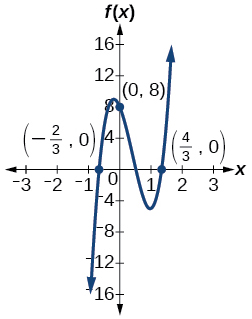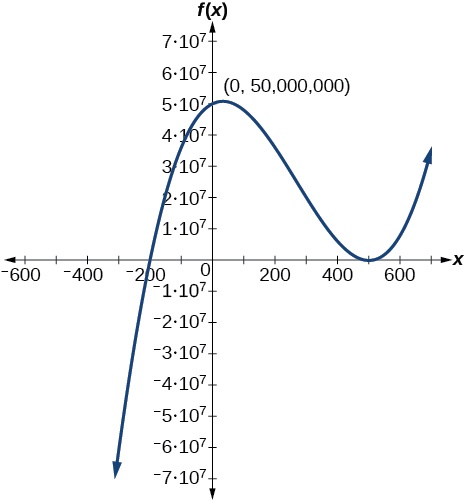$f\left(x\right)={\left(x-500\right)}^{2}\left(x+200\right)$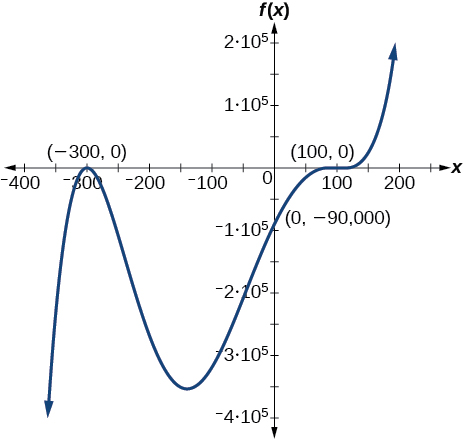## Real-world applications

For the following exercises, write the polynomial function that models the given situation.

A rectangle has a length of 10 units and a width of 8 units. Squares of $\text{\hspace{0.17em}}x\text{\hspace{0.17em}}$ by $\text{\hspace{0.17em}}x\text{\hspace{0.17em}}$ units are cut out of each corner, and then the sides are folded up to create an open box. Express the volume of the box as a polynomial function in terms of $\text{\hspace{0.17em}}x.$

$f\left(x\right)=4{x}^{3}-36{x}^{2}+80x$

Consider the same rectangle of the preceding problem. Squares of $\text{\hspace{0.17em}}2x\text{\hspace{0.17em}}$ by $\text{\hspace{0.17em}}2x\text{\hspace{0.17em}}$ units are cut out of each corner. Express the volume of the box as a polynomial in terms of $\text{\hspace{0.17em}}x.$

A square has sides of 12 units. Squares by units are cut out of each corner, and then the sides are folded up to create an open box. Express the volume of the box as a function in terms of $\text{\hspace{0.17em}}x.$

$f\left(x\right)=4{x}^{3}-36{x}^{2}+60x+100$

A cylinder has a radius of $\text{\hspace{0.17em}}x+2\text{\hspace{0.17em}}$ units and a height of 3 units greater. Express the volume of the cylinder as a polynomial function.

A right circular cone has a radius of $\text{\hspace{0.17em}}3x+6\text{\hspace{0.17em}}$ and a height 3 units less. Express the volume of the cone as a polynomial function. The volume of a cone is $\text{\hspace{0.17em}}V=\frac{1}{3}\pi {r}^{2}h\text{\hspace{0.17em}}$ for radius $\text{\hspace{0.17em}}r\text{\hspace{0.17em}}$ and height $\text{\hspace{0.17em}}h.$

$f\left(x\right)=\pi \left(9{x}^{3}+45{x}^{2}+72x+36\right)$

#### Questions & Answers

write down the polynomial function with root 1/3,2,-3 with solution
if A and B are subspaces of V prove that (A+B)/B=A/(A-B)
write down the value of each of the following in surd form a)cos(-65°) b)sin(-180°)c)tan(225°)d)tan(135°)
Prove that (sinA/1-cosA - 1-cosA/sinA) (cosA/1-sinA - 1-sinA/cosA) = 4
what is the answer to dividing negative index
In a triangle ABC prove that. (b+c)cosA+(c+a)cosB+(a+b)cisC=a+b+c.
give me the waec 2019 questions
the polar co-ordinate of the point (-1, -1)
prove the identites sin x ( 1+ tan x )+ cos x ( 1+ cot x )= sec x + cosec x
tanh`(x-iy) =A+iB, find A and B
B=Ai-itan(hx-hiy)
Rukmini
what is the addition of 101011 with 101010
If those numbers are binary, it's 1010101. If they are base 10, it's 202021.
Jack
extra power 4 minus 5 x cube + 7 x square minus 5 x + 1 equal to zero
the gradient function of a curve is 2x+4 and the curve passes through point (1,4) find the equation of the curve
1+cos²A/cos²A=2cosec²A-1
test for convergence the series 1+x/2+2!/9x3ByByByByBy